# Flow measurement for inaccessible conductive fluids - Lorentz force velocimetry

Used in flow meters for measurement of velocity of fluids. Using the principle of electromagnetic flow measurement, which does not need contact with the fluid measured. The measurement of flow velocities in hot and aggressive liquids can be done apart from measuring the velocity of solid materials and detection of structural micro-defects in them.

Also known as Lorentz force flowmeter, It measures the bulk Lorentz Force from interaction between the metallic fluid And Applied Magnetic field. For this the Magnitude of length of the magnetic field Is same as the channel dimension.

# Principle of operation of Lorentz force velocimetry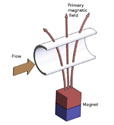An electrically conductive fluid is exposed to a magnetic field.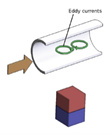The magnetic field induces eddy currents inside the fluid according to faradays law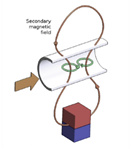The eddy currents give rise to a secondary magnetic field according to Amperes law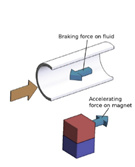A opposing force to the direction of the flow called the braking force is developed ,which is proportional to the accelerating force on the magnet.

The measure of the accelerating force is a direct measure of the fluid velocity.

# Factors affecting the measurement of fluid velocity

Eddy current strength that is the primary cause for the measurement depended on

1. the conductivity of the metallic fluid.
2. Relative movement between the fluid and the permanent magnet
3. Magnitude of the magnetic field
4. Direction of the magnetic field with respect to the flow direction.

# Designs of Lorentz force flowmeters.

Static flowmeters: Magnet system is at rest and flow rate is the measures of force acting on it. Rotary flowmeters: Magnets are arranged on a rotating wheel and the spinning velocity is a measure of the flow velocity. Depending on the magnetic field direction, the flow meters are classified as

1. Longitudinal Lorentz force flowmeters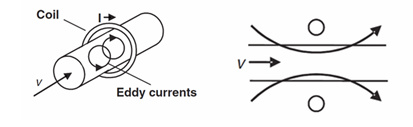2. Transverse Lorentz Force Flowmeters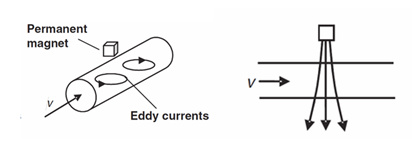Rotary LFF is either freely rotating permanent magnet or an array of magnets mounted on a flywheel which is magnetized perpendicularly to the axle it is mounted on. When placed adjacent to a pipe carrying an electrically conducting fluid the flywheel rotates such that the driving torque due to the eddy currents induced by the flow is balanced by the braking torque induced by the rotation itself. The rotation rate is proportional to the flow velocity and inversely proportional to the distance between the magnet and the pipe. Here either the torque on the magnet system or the angular velocity at which the wheel spins is measured.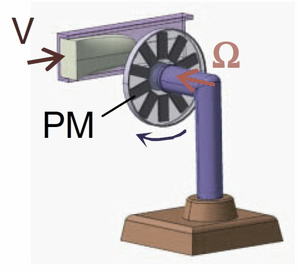# Applications of Lorentz force flowmeters

## 1. Flow rate of High Conducting liquid or solid metals

The effect on the magnetic system is measured using a strain gauge whose deflection is recorded using a interferometer. A deflection of less than 0.1 nanometer can be detected.

## 2. Flow rate of Low Conducting Electrolytic solution or glass melts

For fluids with low conductivity flowing within opaque structures at high temperatures and radiation, nonconductive flow meters are necessary. In such cases where the conductivity is low and the magnetic force is feeble, highly sensitive and precise force measurement system has to be developed. This is in the developmental stages using weights hung like a pendulum with high power magnets acting as the weight, the movement of these pendulum is recorded using an interferometer. To reduce environmental effects, dummy weights are used as compensation. Having them in a array amplifies the small measurement possible with low conductivity fluids.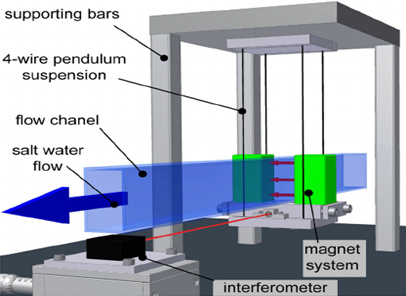## 3. Electrical conductivity of solid and molten metals - Lorentz force sigmometry

Lorentz force sigmometry is the technique to determine density, viscosity, thermal conductivity, electrical conductivity, surface tension of the metallic fluids. Electrical conductivity is a function of mass flow rate and Lorentz force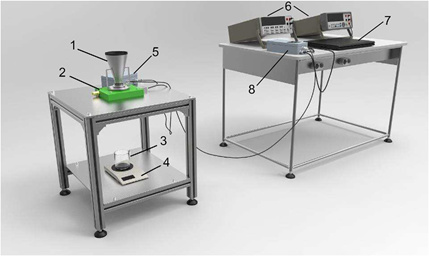Components : 1: filling funnel; 2: Halbach magnet and force sensor; 3: collecting vessel; 4: electronic scale; 5: amplifier and converter; 6: voltmeters; 7: computer; 8: transformer and converter.

## 4. Fluid velocity when conductivity is unknown.

Time of flight Lorentz force velocimetry is used when the fluid under measurement has an unknown conductivity. It is designed to be independent of the fluid conductivity. It is made of one or two coils placed around a circular pipe. The forces produced on each coil are recorded in time as the liquid metal flows through the pipe and cross-correlated thus the forces can be used to determine the flowrate. Very useful in metallurgical industry where the conductivity of the fluid is almost always unknown.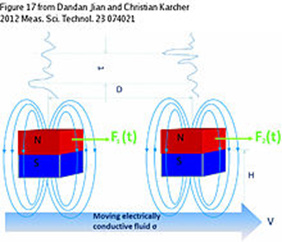For further reading go through this white paper

## 5. Defect analysis

Lorentz eddy current test is a modification of the traditional AC eddy current test. Basic principle of eddy current test : A sample with defect will have nonuniform electrical conductivity, disturbances in the eddy current path.

Difference between eddy current test and Lorentz eddy current test

Parameters Eddy current test Lorentz eddy current test
Supply used AC magnetic field DC magnetic field
Working mechanism Alternating (AC) magnetic field induces eddy currents inside the material under test Eddy currents are generated by providing the relative motion between the conductor under test and a permanent magnet
Detection of eddy current perturbance Change in the impedance of the supply AC coil due to the eddy current. Lorentz force fluctuation on the magnet
Defect identification Immediate vicinity of the surface of a material ( 1mm) Deep and relatively fast testing

# Advantages of Lorentz Force Flowmeter

• Non-contact techniques of flow rate measurement.
• Used for aggressive and high-temperature fluids like liquid metals.
• Independent of flow’s inhomogeneities and zones of turbulence.
• Can also be used in open channel measurement

# Limitations of Lorentz Force Flowmeter

• Temperature control of measurement system as magnetic field is dependent on temperature. ( Above curie temperature, the magnetic property is lost)
• Permanent magnet’s dimensions determines the measurement zone
• Rapid decay of the magnetic fields give rise to tiny forces on the magnet system
• Liquid level should be controlled for measurement in open channel.